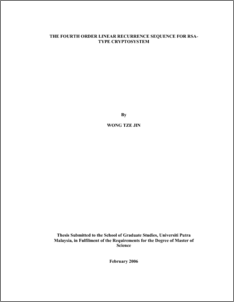# The Fourth Order Linear Recurrence Sequence For RSA Type Cryptosystem

## Citation

Wong, Tze Jin (2006) The Fourth Order Linear Recurrence Sequence For RSA Type Cryptosystem. Masters thesis, Universiti Putra Malaysia.

## Abstract

A cryptosystem is derived from the fourth order linear recurrence relations analogous to the RSA cryptosystem which is based on Lucas sequences. The fourth order linear recurrence sequence is a sequence of integers −1 −2 −3 −4 = − + − n n n n n V PV QV RV SV , where P, Q, R and S are coefficients in quartic polynomial, 0 4 3 2 x − Px + Qx − Rx + S = .The factorization of the quartic polynomial modulo p can be classified into five major types. We define the cyclic structure for every types. Then, we can generate the Euler totient function from the cyclic structure of every types of the quartic polynomial.We have some properties of the sequence which are straightforward consequences of the definition. Then, we are able to define the composition and inverse of fourth order linear recurrence sequence. From cycles and totient, we know the quartic polynomial can be factorized into five major types, that is t, t[3,1], t[2,1], t and t. We sketch an algorithm to compute the type of a quartic polynomial in Fp[x], where p is any prime number. In this quartic cryptosystem, we have two large secret primes p and q, the product N of which is part of the encryption key. The encryption key is (e, N) where e is relatively prime toΦ(N) , which are analogous to Euler-φ function, to cover all possible cases. The decoding key, d is inverse e modulo Φ(N).For quartic cryptosystem, (P,Q,R) constitutes the message, and ( , , ) 1 2 3 C C C constitutes the ciphertext. In decoding, we are given the function ( ) g x = x −C x +C x −C x + but not ( ) 1 4 3 2 f x = x − Px + Qx − Rx + , and so we have to deduce the type of f in order to apply the algorithm correctlyPreview
PDF
549098_ipm_2006_1_abstrak_je__dh_pdf_.pdfView Item